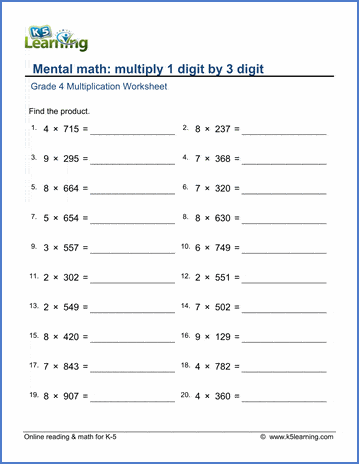# Multiply 1-digit by 3-digit numbers

## Mental multiplication worksheets: multiply 1-digit by 3-digit numbers

Below are six versions of our grade 4 math worksheet on multiplying 1-digit by 3-digit numbers (up to 999). Students should try to figure out the answers mentally, regrouping in their heads, without writing down intermediary steps. Challenging, but great practice!  These worksheets are pdf files.## More multiplication worksheets

Find all of our multiplication worksheets, from basic multiplication facts to multiplying multi-digit whole numbers in columns.

## What is K5?

K5 Learning offers reading and math worksheets, workbooks and an online reading and math program for kids in kindergarten to grade 5.  We help your children build good study habits and excel in school.